Pandas is the most widely used tool for data munging. It contains high-level data structures and manipulation tools designed to make data analysis fast and easy.

In this post, I am going to discuss the most frequently used pandas features. I will be using olive oil data set for this tutorial, you can download the data set from this page (scroll down to data section). Apart from serving as a quick reference, I hope this post will help you to quickly start extracting value from Pandas. So lets get started!

1) Loading Data

“The Olive Oils data set has eight explanatory variables (levels of fatty acids in the oils) and nine classes(areas of Italy)”. For more information you can check my Ipython notebook.

I am importing numpy, pandas and matplotlib modules.

```%matplotlib inline
import numpy as np
import matplotlib.pyplot as plt
import pandas as pd ```

I am using pd.read_csv to load olive oil data set. Function head returns the first n rows of ‘olive.csv’. Here I am returning the first 5 rows.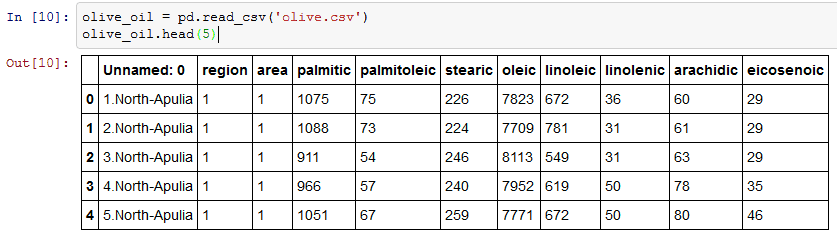2) Rename Function

I am going to rename the first column (‘Unnamed: 0) to ‘area_Idili’.Rename function as an argument it takes a dictionary of column names that should be renamed as keys(olive_oil.columns) and the new title(‘area_Idili’) to be the value. Olive_oil.columns will return the column names. inplace = True is used in case you want to modify the existing DataFrame.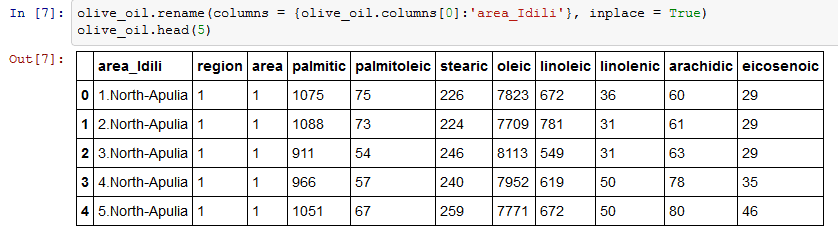3) Map

One thing that I want to do is to clean the area_Idli column and remove the numbers. I am using map object to perform this operation. Map property applies changes to every element of a column. I am applying split function to column area_idili.  Split function returns a list, and -1 returns the last element of the list. A detailed explanation of lambda is given here.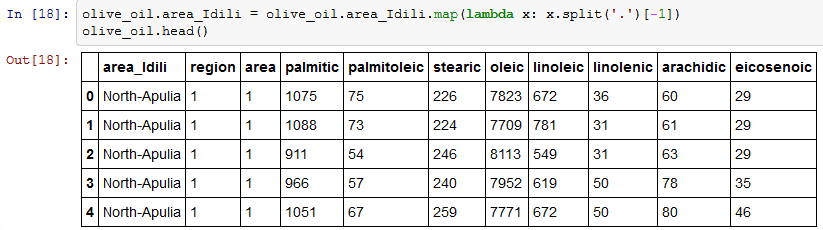See how split function works: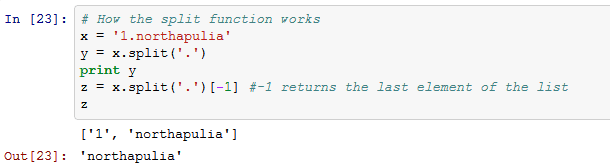4) Apply and Apply Map

I have a list of acids called acidlist. Apply is a pretty flexible function, it applies a function along any axis of the DataFrame. I will be using applyfunction to divide each value of the acid by 100.

list_of_acids =[‘palmitic’, ‘palmitoleic’, ‘stearic’, ‘oleic’, ‘linoleic’, ‘linolenic’, ‘arachidic’, ‘eicosenoic’]

``` df = olive_oil[list_of_acids].apply (lambda x: x/100.00)
df.head (5) ```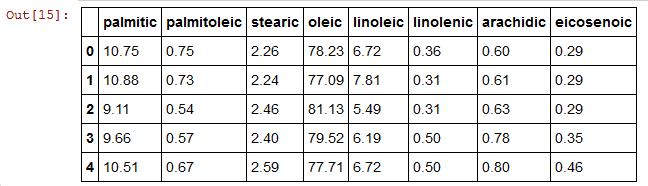Similar to apply, apply map function works element-wise on a DataFrame.

Summing up, apply works on a row/column basis of a DataFrame,applymap works element-wise on a DataFrame, and map works element-wise on a Series.

5) Shape and Columns

Shape property will return a tuple of the shape of the data frame.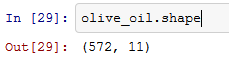olive_oil.columns will give you the column values.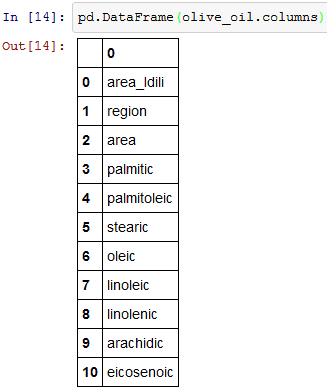6) Unique function

Olive_oil.region.unique() will return unique entries in region column, there are three unique regions (1,2,3). I am applying the same unique property to area column, there are 9 unique areas.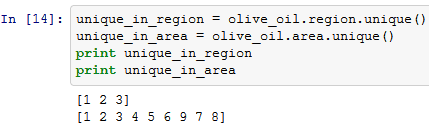7) Cross Tab

Cross Tab computes the simple cross tabulation of two factors. Here I am applying cross tabulation to area and region columns.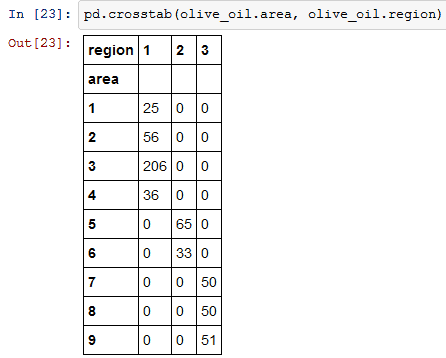8) Accessing Sub data frames

The syntax for indexing multiple columns is given below.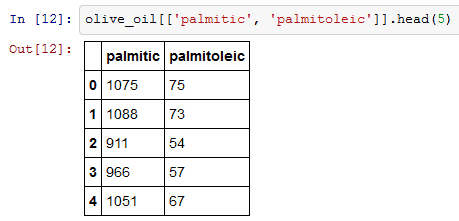To index a single column you can use olive_oil[‘palmitic’] orolive_oil.palmitic.

9) Plotting

plt.hist(olive_oil.palmitic). You can plot histogram using plt.hist function.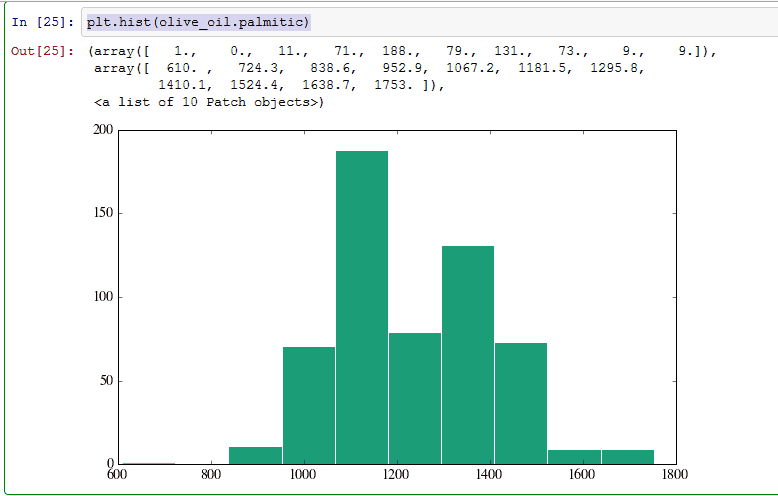You can also generate subplots of pandas data frame.  Here I am generating 4 different subplots for palmitic and  linolenic columns.  You can set the  size of the figure using figsize object, nrows and ncols are nothing but  the number of columns and rows.10) Groupby and Statistics

Groupby groups the data into 3 parts(region 1, 2 and 3). The functiongroupby gives dictionary like object. Here I am grouping by regions [olive_oil.groupby(‘region’)].

I am applying describe on the group, describe takes any data frame and compute statistics on it. This is the quick way of getting statistics by group of any data frame.You can also calculate standard deviation of the region_groupby  using olive_oil.groupby(‘region’).std()11) Aggregate function

Aggregate function takes a function as an argument and applies the function to columns in the groupby sub dataframe. I am applying np.mean(computes mean) on all  three regions.12) Join

I am renaming ol mean and olstd columns.

In[ 34]: list_of_acids =[‘palmitic’, ‘palmitoleic’, ‘stearic’, ‘oleic’, ‘linoleic’, ‘linolenic’, ‘arachidic’, ‘eicosenoic’]Pandas can do general merges. When we do that along an index, it’s called a join. Here I make two sub-data frames and join them on the common region index.13) Masking

You can also mask a particular part of the data frame.

olive_oil.eicosenoic < 0.05  will check if each value in column eicosenoic is less than 0.05, if the value is less than 0.05 then it will return true, else it will return false.

In : eico=(olive_oil.eicosenoic < 0.05)14) Handling Missing Values

Missing data is common in most data analysis applications. I find drop na and fill na function very useful while handling missing data.

I am creating a new data frame.The dropna can used to drop rows or columns with missing data (None). By default, it drops all rows with any missing entry.fillna can be used to fill missing data (None). First, I am creating a data frame with a single column.I am using fillna replaces the missing values with the mean of DataFrame(data).Conclusion

These are some of the important functions I use frequently while cleaning data. I highly recommend Wes Micknney’s Python for Data Analysis book for learning pandas. Is there any other important pandas function that I missed?Manu Jeevan is a  Data science and Analytics blogger at BigDataExaminer, where he writes about Data Science, Python and Digital analytics.

Photo credit: Smithsonian’s National Zoo / Foter